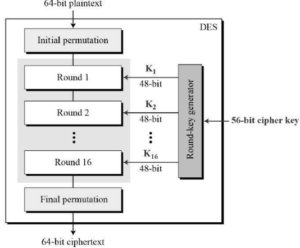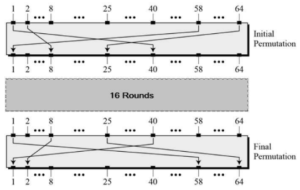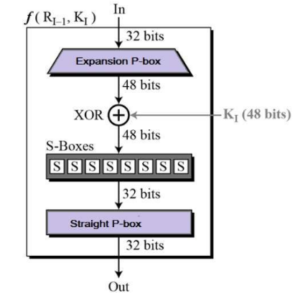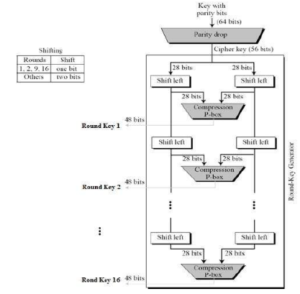# Data Encryption Standard

The Data Encryption Standard (DES) is a symmetric-key block cipher published by the National Institute of Standards and Technology (NIST).

DES is an implementation of a Feistel Cipher. It uses 16 round Feistel structure. The block size is 64-bit. Though, key length is 64-bit, DES has an effective key length of 56 bits, since 8 of the 64 bits of the key are not used by the encryption algorithm (function as check bits only). General Structure of DES is depicted in the following illustration −Since DES is based on the Feistel Cipher, all that is required to specify DES is −

• Round function
• Key schedule
• Any additional processing − Initial and final permutation

## Initial and Final Permutation

The initial and final permutations are straight Permutation boxes (P-boxes) that are inverses of each other. They have no cryptography significance in DES. The initial and final permutations are shown as follows −## Round Function

The heart of this cipher is the DES function, f. The DES function applies a 48-bit key to the rightmost 32 bits to produce a 32-bit output.Expansion Permutation Box − Since right input is 32-bit and round key is a 48-bit, we first need to expand right input to 48 bits.

• XOR (Whitener). − After the expansion permutation, DES does XOR operation on the expanded right section and the round key. The round key is used only in this operation.
• Substitution Boxes. − The S-boxes carry out the real mixing (confusion). DES uses 8 S-boxes, each with a 6-bit input and a 4-bit output.

## Key Generation

The round-key generator creates sixteen 48-bit keys out of a 56-bit cipher key. The process of key generation is depicted in the following illustration −